# Game Makers and programming language chat thread

4 replies to this topic

### #1LikeLike888

LikeLike888

Spicy food lover!!

•• Members
•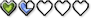• Real Name:Jason
• Gender:Male
• Location:North America

Posted 13 September 2021 - 12:30 PM

Here is an example of if relative working GML (Game Maker Language) example:

```Create Event
hp = 12; //variable hp is equal to 12
hp_divided_by = 1; //will be used to help divide (reverse [opposite] of multiply) hp
hp_minus = 0; //will be used to help decrease hp
hp_plus = 0; //will be used to help increase hp
hp_times_by = 1; //will be used to help multiply hp

Step Event
if (hp_times_by <> 1) //if variable hp_times_by is less than 1 or greater than 1
{
hp *= hp_times_by; //variable hp gets multiplied by the amount of whatever variable hp_times_by is equal to
hp_times_by = 1; //variable hp_times_by is equal to 1
}
if (hp_plus <> 0) //if variable hp_plus is less than 0 or greater than 0
{
hp += hp_plus; //variable hp gets increased by whatever variable hp_plus is equal to
hp_plus = 0; //variable hp_plus is equal to 0
}
if (hp_minus <> 0) //if variable hp_plus is less than 0 or greater than 0
{
hp -= hp_minus; //variable hp gets decreased by whatever variable hp_minus is equal to
hp_minus = 0; //variable hp_minus is equal to 0
}
if (hp_divided_by <> 1) //if variable hp_divided_by is less than 1 or greater than 1
{
hp_divided_by != 0; //variable hp_divided_by is not equal to 0 to prevent errors
hp /= hp_divided_by; //variable hp gets divided by whatever variable hp_divided_by is equal to
hp_divided_by = 1; //variable hp_divided_by is now equal to 1
}
if (keyboard_check_pressed(ord("D"))) //if D button is pressed (pushed)
{
hp_divided_by = 2; //variable hp_divided_by is equal to 2
}
if (keyboard_check_pressed(ord("M"))) //if M button is pressed
{
hp_minus = 2; //variable hp_minus is equal to 2
}
if (keyboard_check_pressed(ord("P"))) //if P button is pressed
{
hp_plus = 2; //variable hp_plus is equal to 2
}
if (keyboard_check_pressed(ord("T"))) //if T button is pressed
{
hp_times_by = 2; //variable hp_times_by is equal to 2
}
if (keyboard_check_pressed(ord("Z"))) //if Z button is pressed
{
show_message("x: "+string(x)+"#"+"y: "+string(y)+"#"+"hp: "+string(hp)); //tells x and y position and hp amount # each on a different line of text
}
if (hp_times_by == 2) //if variable hp_times_by is equal to 2
{
x -= 2; //go left by two pixels
}
if (hp_plus == 2) //if variable hp_plus is equal to 2
{
x += 2; //go right by two pixels
}
if (hp_minus == 2) //if variable hp_minus is equal to 2
{
y -= 2; //go up by two pixels
}
if (hp_divided_by == 2) //if variable hp_divided_by is equal to 2
{
y += 2; //go down by two pixels
}
```

• Bagu likes this

### #2P-Tux7

P-Tux7

Apprentice

•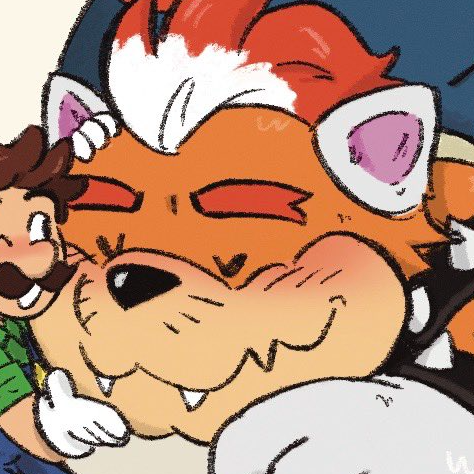• Members
•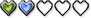Posted 14 September 2021 - 12:07 AM

Game Maker is lamer than ZC because you have to pay for it and also pay for exporting to Linux separately

### #3TheManHimself

TheManHimself

Real Human Bean

•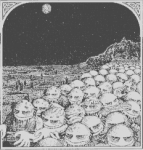• Members
•• Real Name:Shane
• Gender:Male

Posted 15 September 2021 - 01:50 PM

LikeLike88 u r a raconteur.

• Rambly likes this

### #4NoeL

NoeL

Legend

•• Members
•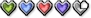• Real Name:Jerram
• Gender:Male

Posted 15 September 2021 - 08:47 PM

I'm not sure what the point of this thread is.

Game Maker is lamer than ZC because you have to pay for it and also pay for exporting to Linux separately

ZC is lamer than Love2D because it's based on Allegro and Allegro sucks.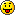### #5Geoffrey

Geoffrey

Chosen One

•• Members
•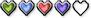• Gender:Unspecified

Posted 11 October 2021 - 03:28 PM

ZC is lamer than Love2D because it's based on Allegro and Allegro sucks.ZC may be lame, but cripples still get married (sometimes).

• Shane likes this

#### 1 user(s) are reading this topic

0 members, 1 guests, 0 anonymous users